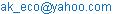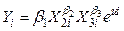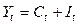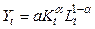International Journal of Economics, Finance and Management Sciences
Volume 5, Issue 2, April 2017, Pages: 98-101

Towards Constructing a Dynamic Model of Economics for Investigating Investment and Production in Bangladesh

Akram Hossain Md.

Department of Economics, Rajshahi University, Rajshahi, BangladeshAkram Hossain Md. Towards Constructing a Dynamic Model of Economics for Investigating Investment and Production in Bangladesh. International Journal of Economics, Finance and Management Sciences. Vol. 5, No. 2, 2017, pp. 98-101. doi:10.11648/j.ijefm.20170502.13

Received: November 24, 2016; Accepted: December 17, 2016; Published: January 26, 2017

Keywords: EPZ, Investment, Consumption, Capital Resources, Elasticity of Capital, the Main Production Funds, Human Resources, Dynamic Economic Model

Contents

1. Introduction

It is a common place idea that the GDP and investments are closely related, and wide attraction of investment can lead to growth in GDP. "Keynesian multiplier shows the relation between investment and economic growth. In Bangladesh it is very essential to attract foreign investment into production of especially industries working in the export processing zone (EPZ). Bangladesh government pursues all possible ways to attract foreign direct and indirect investment. Domestic investment is not up to the requirements. There are huge money remains idle in the banking system. Here one important thing which should be mentioned that private banking system is improving day by day. Bangladesh reaches lower middle income group with a huge burden of unemployment on it’ shoulder. Attraction of domestic as well as foreign direct and indirect investment is very necessary. Investment can lead us to the middle incomecountry earlier than 2030.

Accumulation process is of vital importance. It comprisesinvestment consisting of (a) gross domestic savings as % of GDP, (b) gross domestic investment as % of GDP, and (c) capital inflow as % of GDP (Hollis B, Chenery) . Investment plays an important role in the structural changes in the production of GDP (BurkanovAktam) .) Therefore, the relationship between the GDP and investment and creating models of investment growth is significant. We need to estimatea dynamic economic model for Bangladesh.

The theory of economics can highlight the use of two lines;

a)Models not linked to the real economy, with less specific examples for modeling and experimental uses;

b)Modeling possible directions of economic development of multiple studies of real economic dynamics and coordinated in order to make management decisions.

Type ‘b’ is more difficult as it requires to create models of real economic dynamics. As a result academic economists were forced to build abstract models. Now-a-days growth of GDP not only attracts domestic investment but also attracts foreign investment (both directly and indirectly). There are huge number of countries and financial institutions highly capable of providing us with necessary amount of foreign capital in the form of investment. To use our investible fund foreign direct and indirect investment can play a vital role. When domestic entrepreneurs will see the working of foreign investment, they will be encouraged to invest their money which remains idle. Once this process is started GDP will increase. This model is estimated in Bangladesh. Wherethe growth of GDP is near about 7%.

2. Literature Review

Economist scientists have created models with various aspects of the connection between GDP and investment levels. Scientific and practical significance of these models is that the connection between investment and different aspects of GDP have been proven in practice. For example" the relationship between increase in the volume of investment and growth rate of GDP" (J.M Keynes model)  "the relationship between increase in investment and growth of macroeconomic indicators (Cobb-Douglas function) are proven to have practical significance. Capital formation by increasing investment is another crucial and strategic determinant of the growth of GDP. Professor H.W. Singer  has visualized the process of economic growth in terms of the following determinants:

D=SP-r(1)

Where,

D=Rate of economic development;

S=The rate of net saving;

P=productivity of the new investment per unit of capital;

r=Rate of annual increase of population.

Equation (1) is of great importance in the sense that economic development is closely related with therate of saving as well as with the productivity of the investment. Increase in net saving will increase investment which in turn increases GDP.

Along with capital formation, skill-formation is also an essential prerequisite of economic growth. The history of allthe advanced countries bears testimony to the fact that their periods of expansion have always been characterized by a high rate of capital formation. Economists have created models with various aspects of the connection between GDP and investment Domar model clearly shows that investment determines the actual level of income through the Keynesian multiplier process (Hywel Jones) 

Y=(1/s)I(2)

Here,

Y-total income,

S-marginal propensity to save,

I-investment

Growth rate of GDP when increases it attracts domestic investment, foreign direct and indirect investment.. R. Solow who created the first model of economic dynamics, saw it as the complex function of Gross National Product (GNP) and capital resources. Studying GDP by dividing it into two components, namely consumption and investments was carried out for the first time by academic economist J.M. Keynes (Keynes, 1993). During the scientific experiment, Keynes suggested to study the GDP by dividing it into two parts; consumption and savings. Also, he concluded that as the savings are redirected into production as investment, this will lead to growth of real income. In 1928, C. Cobb and P. Douglas created economic growth model where GDP is the function of the relationship between physical capital and human resources (Robert J. Barro, Xavier Sala-I-Martin, 2003) . The model of production function looks as follows:(3)

Here,

Y-production, GDP

X2-human resources employed to create the GDP;

X3-capital resources exploited to create the GDP

u-stochastic parameter, function error;

e-natural logarithmic base.

Specific features of Cobb-Douglas model:

β2 is the elasticity of production relative to workforce, and when the capital was taken as a constant factor, shows the percent change in the production as a result of 1% increase in labor force;

β3shows the percentage change in production as a result of 1% increase in capital resources while labor force is constant

β23 the sum of the two shows the change in production as a result of proportional change in production factors. If this sum is equal to 1 then it clearly shows constant returns to scale. If the total of the sum is smaller than 1 then the result is diminishing.

All variables used in the Cobb-Douglas model are considered stochastic with 10% probability according to Dicky-Fuller test. As is known, in accordance with the method of OLS, for variable coefficients to be a real, model errors should have normal distribution as well as exogenous variables and model error should have no correlation and should fulfill other conditions. O. Blancharda professor at the University of Massachusetts in the United States has proven the connection between investment and GDP using a model (Blanchard, 2003). This is a very simple model (I=). where investments are not significantly sensitive to changes in production. In O. Blanchard’s model increase in investment with the purpose of increasing production which results in increase in GDP is not reflected. According to G. Mankiw’s conclusion (Mankiw, 2007) , if we take into account that national savings equal to Y-C-G, GDP and investment will look as follows:

Y-C-G=I+NX=S(4)

Here,

Y-GDP;

C-consumption;

G-government expenditure;

I-investment;

NX-net export;

S=national saving;

G, Mankiw considers net exports to be equal to net capital expenditures and offers the following equation (Mankiw, 2007):

S=I+NCO(5)

He ultimately concludes that the national savings should be equal to the sum of the domestic investment and net capital expenditure. S.V Chepel achieved the following important scientific results in the process of applying the theoretical model of attracting direct foreign investments into the national economy in practice (Chepel, 2014)  GDP deflator’s decline of 10 percentage points in analyzed countries, the volume of direct foreign investments in GDP increased 0.8 percentage points. The decrease in the rate of inflation increase in direct foreign investments in the share of GDP ( 2007 GDP deflator cut inflation at the level of 2000) increased by 23 percentage points in this period, the share of direct foreign investments in GDP to increase by 0.8 percent to 3.2 percent). The development of the banking system, involved in the improvement of the quality indicators of the national economy will increase the volume of direct foreign investments. In foreign direct investment attracting model, regulatory changes regarding the investment should be considered. The reason for this is that the decline in investment does not allow us to maintain the stability.

3. Methodology

If current GDP is taken as Yt, consumption-Ct, investment-It, then according to the conclusion of Keynes the following formula will be true(6)

Volume of consumer demand consists of two indicators-consumption determined by minimum hygiene standards and income growth as a result of additional parts Keynes suggested that the second part is reflected as a percentage of GDP in the previous period: cYt-1

c=share of consumption in GDP

In this case, this year’s consumer demand constitutes linear function of last year’s GDP:

Ct=C+cYt-1 (7)

If we put it into equation 6 we get

Yt=C+cYt-1+It(8)

Here,

c-share of consumption in GDP.

If investments are taken as constant, then we will have first order autoregressive equation. Propensity to consume affects GDP growth rate (c-share). As it is autoregressive model, we can illustrate different trajectories of GDP growth by setting initial condition and changing the value of propensity to consume. We can create a closed model by combining Keynesian model and Cobb-Douglas Production function (A. Koutsoyiannis)  and by defining different parameters we can estimate different growth projections of the economy. In Solow model, GDP is determined with the help of Cobb-Douglas production function as follows:(9)

In a given time (t) GDP (Yt) consists of investments (It) and consumption (Ct)

Yt=I t+ Ct(10)

Here the focus is on investment rather than consumption and share of GDP which is expected to be allocated for investments can be given as propensity to save-ρ;

It=ρ Yt(11)

ρ=marginal propensity to save

As is known, attraction of investments will lead to the growth of production funds for the next year Kt+1 which is determined through old funds Kt by taking into account the share of funds from last year’s production µ;

Kt+1=(1-µ) Kt+It(12)

µ=the share of funds from last year’s production

If the number of labor in the economy are labeled as Lt+1, taking into account annual growth rate of labor force (γ), it can be calculated through current number of labor force:

Lt+1=(1+γ)Lt(13)

γ=annual growth rate of labor

GDP, investments and other parameters can be calculated taking into account next year’s production funds and number of labor. Equations (9)-(13) are mathematic expression of Solow model. We have estimated the models (9)-(13) for Bangladesh using simple OLS method. We have used data from two sources World Development Indicators and http://www.imf.org/external/pubs/ft/weo/2016/02/weodata/weoselser.aspx?c=513&t=1,. We have used data for the period of 1996-2014

4. Result

To estimate the model we have made the model linear by taking logarithm. We obtaindata on capital and labor for the period 1996-2014 for Bangladesh from World Development Indicators and IMF sources.

We have estimated the model  using the method of OLS. Following are the results.

Y=18.53K1.08L-1.06(14)

Table 1. Model Results.

 Variables Parameters p-value K 1.08 0.000 L -1.06 0.000 Cons. 18.53 0.000 R2 0.99 F 5051.74 Prob>F 0.000

This model shows capital resources elasticity 1.08 and of the labor-1.06. Summation of the two shows decreasing returns to scale. It is an important question to think about the results given above. Presently in Bangladesh per capita GDP growth rate is near about 7%. It is due to the fact of very high level of efficiency factor (18.53)

The labor elasticity is-1.06. It is probably due to huge unemployment problem in Bangladesh.

That the constant or efficiency factor of the model is very high. It is 18.53. It means the high efficiency of production performance in Bangladesh during 1996-2014.

All the results are statistically significant.

It is important to describe other aspects of this model. Production function itself not enough to construct the model of dynamic economics. Because GDP is divided into consumption and savings, calculating the share of GDP allocated for investments ρ is necessary. Here it is 0.17: It is quiet significant that in Bangladesh saving is 17% of GDP

It=0.17Qt(15)

Investments lead to rise in the main funds of the following year Kt+1

Kt+1=0.054Kt+It   (16)

Capital depreciation is 0.054 which is reasonable.

Annual rise in labor force 2.01, therefore number of labor in economy is calculated through current number of labor Lt

Lt+1=2.01Lt(17)

2.01 is also reasonable for Bangladesh.

All the results are statistically significant. One of the most important outcome of this researchis that in Bangladesh producers are very efficient.

5. Conclusion

This model can be implemented in economic development of sectors and as well as used in working out suggestions and conclusions in further development of a particular sector. The results of the estimation show very important aspects of Bangladeshi economy. It was to me a difficult question how Bangladesh is growing at the rate of about 7%, how it is reaching a lower middle income country. The results of the research work give the answers to the questions. The efficiency factor of our producers from agriculture to everywhere is very high (18.53).According to the results of the work some simple suggestions can be made. Training and other efficiency increasing facilities need be taken for further development of Bangladesh. Bangladesh is likely to be a rich country by 2041.

References

1. Hollis B, Chenery (1979). Structural change and development policy.
2. BurkhanovAktam (2016). Econometric model of attracting investment into production in Uzbek industry: volume 5, version 1, IOSR-JEF Sep-Oct 2016.
3. Keynes J. M (1993). Selected works. -M. Economics. -pp. 224-518.
4. Singer, H. W. (1949). Economic progress in underdeveloped countries. Social Research, 1-11.
5. Jones, H. G. (1975). An introduction to modern theories of economic growth. London: Nelson, pp, 62-65.
6. Robert J. Barro, Xavier Sala-I-Martin (2003): Economic growth, New York: McGraw-Hill, Inc. -pp 158-159.
7. Blanchard, Olivier (2003). Macroeconomics. Third Edition. Pearson Education Inc; Prentice Hall p, 52.
8. Mankiw. N. Gregory (2007). Principles of Macroeconomics, 4th edition, Thomson South-Western-p 346-3 South-Western. -pp. 346-347.
9. Chepel. S. V. (2014). Systematic analysis and modeling of perspective of sustainable development of national economy of Uzbekistan, Monograph,-Tashkent: IFMR, -pp 120-122.
10. Koutsoyiannis, A. (1985). Modern Microeconomics, 2nd edition.
11. Industry of Uzbekstan. -Tashkent: State Statistics Committee of the republic of Uzbekstn, 2006-2015.
12. World Development Indicator. Source Internet.
13. IMF (http://www.imf.org/external/pubs/ft/weo/2016/02/weodata/weoselser.aspx?c=513&t=1.)

 Contents 1. 2. 3. 4. 5.
Article ToolsAbstractPDF(157K)